# [Algorithms II] Week 3-2 Radix Sorts

This week: string sort.

# 1. Strings in Java

### char data type

• char in C

8-bit integer, 256 characters, 7-bit ASCII code

• char in Java

16-bit Unicode

### String data type

`String`: immutable sequence of characters
operations: lengthe, ith char, substring, concatenate

implementation: using a `char[]`, maintain a `length` and an `offset.` ⇒ substring methode is O(1) time.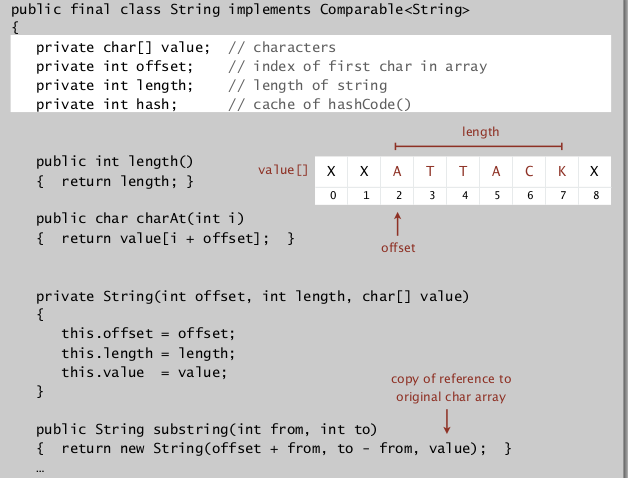### StringBuilder data type

`StringBuilder`: mutable data type.
implementation: using a resizing `char[]` array (ArrayList).
⇒ contat in (amortized) constant time, substring in linear time!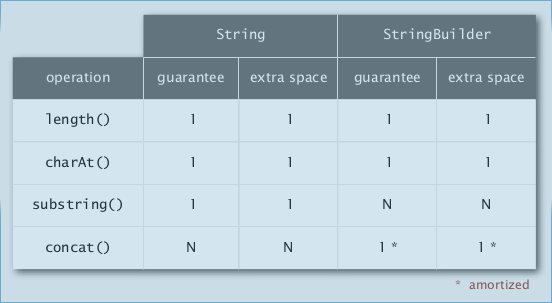ex.

• reverse a string: linear using StringBuilder, quad using String.
• form an array of suffixes: quad (time&space) using StringBuilder, linear (time&space) using String.Longest Common Prefix:
runs in linear/subinear time ⇒ `compareTo()` for strings takes (sub)linear time!

### Alphabet

alphabet different for different type of string (ex. binary numbers, DNA, ...)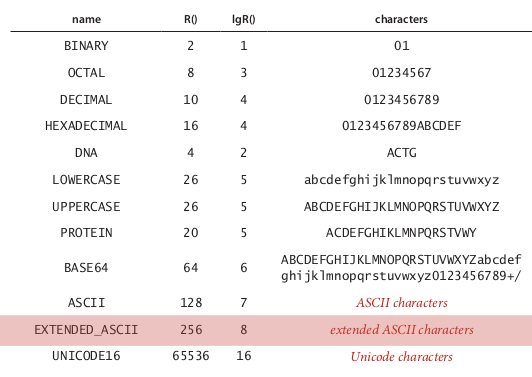def. Radix `R` is number of digits in alphabet.

# 2. Key-Indexed Counting

review of compare-based sorting algorithms:lower-bound for compare-based algorithms: ~NlgN (=Lg(N!))
⇒ goal: do better by avoiding using compares.

### Key-indexed counting

is an algorithm to sort by character(ex. sort array of string by their 1st character).
Assumption: keys are integers between 0 and R-1 (<<N, small integer).
⇒ use keys as array index, to sort an array of N integers between 0 and R-1.

[algo]

• count freq of each key index (of size R+1)
• using count array, compute a cumulated freq (cumsum of `count[]`)
• the `cumsum[]` array stores the index range of each key index:

index range of key-i in sorted array is `[cumsum[i], cumsum[i+1]]`

• then get the sorted array by going through the array and using cumsum[] array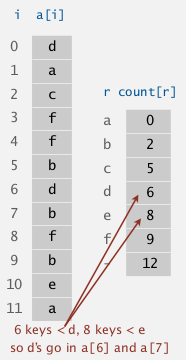```public void keyIndexCounting(int[] a, int R){// entries in a[] are in range [0,R-1]
int N = a.length;
int[] count = new int[R+1]; // count[i] = freq of key i-1, count = 0
for(int ai:a)
count[ai+1]++;
int[] cumsum = count; //cumsum = cumulated freq
for(int i=0;i<R;i++)
cumsum[i+1] += cumsum[i];
// the ranges of key i in sorted array should be [cumsum[i], cumsum[i+1]]
int[] aux = new int[N];
for(int ai:a)
aux[cumsum[ai]++] = ai;
a = aux;
}
```

### analysis

• running time: linear in time and in space.
• stable sorting: that's why we need the cumsum and aux array...

LSD = least significant digit (for string sorting)
(assume strings all of same length)
idea:

• consider chars from right to left
• sort using dth character as key (using key-indexed counting)

important: the key-indexed counting should be stable.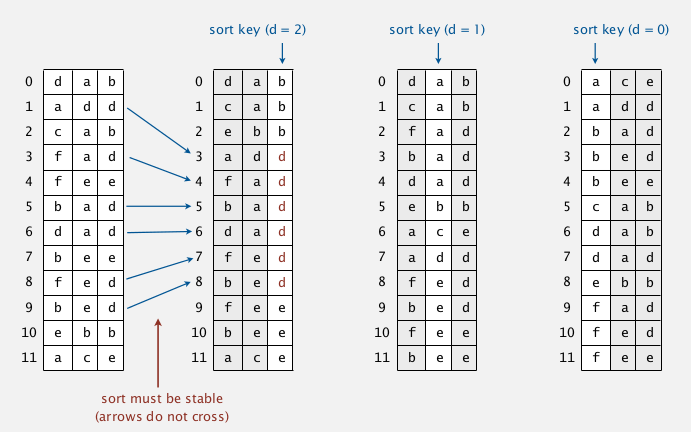### analysis

time: W * N (W=length of string)

correctness:
prop. LSD sorting works
pf. by induction on i
prove that: after pass i, strings are sorted by last i characters.

### implementation

```public static LSDsort(String[] a, int W){//W=fixed width
int R = 256;//for ASCII chars
int N = a.length;
String[] aux = new String[N];
for(int d=W-1;d>=0;d--){//W passes
//key-indexed counting at digit d
int count = new int[R+1];
for(String s:a)
count[s.charAt[d]+1]++;
for(int i=0;i<N;i++)
count[i+1] += count[i];//count = cumsum(count), range of each key
for(String s:a)
aux[s.charAt[d]++]=s;//put each key in right place
for(int i=0;i<n;i++)
a[i]=aux[i];
}
}
```
• if keys are binary numbers → break into bit characters then apply LSD.
• if not fixed length → some fix..
• sort 1 million 32-bit integers? (Google/Obama interview) ⇒ LSD string sort~

most-significant-digit first (from left to right)
Idea.

• partition array into R pieces according to first character (the count[] array is the partition)
• recursively sort each subarrays

variable length: end-of-string are treated as before any char### implementation

```private static void sort(String[] a, String[] aux, int lo, int hi, int d){
if(hi<=lo) return;
//sort by dth character
int count = new int[R+2];// R+2 as we are taking account into the end-of-strings!!
for(int i=lo;i<=hi;i++) count[ a[i].charAt(d)+2 ]++;//charAt(end-of-string)=-1
for(int i=0;i<R;i++) count[i+1] += count[i]; //cumsum
for(int i=lo;i<=hi;i++) aux[count[a[i].charAt(d)+1]++] = a[i];
for(int i=lo;i<=hi;i++) a[i] = aux[i-lo];// attention: aux is filled from index 0 instead of lo
//recursively sort each subarray (R subarrays in total, ranges stored in count[])
for(int r=0;r<R;r++)
sort(a, aux,lo+count[r], lo+count[r+1]-1, d+1);
}
```

### analysis

• can recycle `aux[]`, but not `count[]`.
• too slow for small subarrays (if len(subarray)<<R)
• huge nb of subarrays because of recursion

improvement ⇒ cutoff to insertion sort...

characteristics of MSD sort:
examines just enough chars to sort.
→ can be sublinear in N.### MSD vs. quicksort

• random access of memory (cache inefficient)
• too many instructions in inner loop
• extra space for count[]
• extra space for aux[]

• NlgN nb of string compares
• has to rescan many chars for keys with long prefix matches

⇒ combine benefits of qsort and MSD.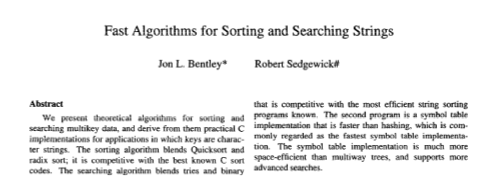idea: do 3-way partition by the dth character.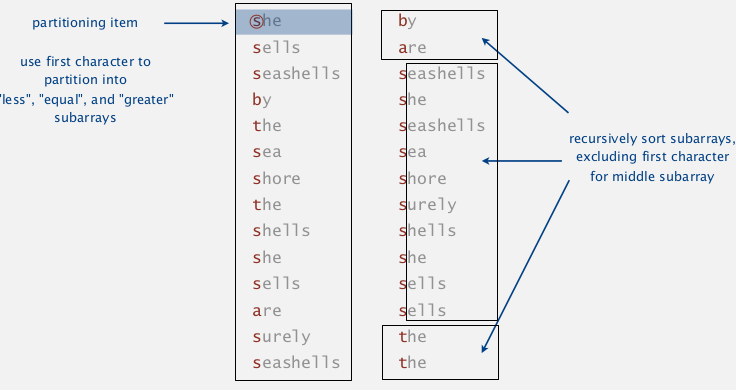• less overhead than R-way partitioning for MSD
• do not re-examine chars equal to the partitioning char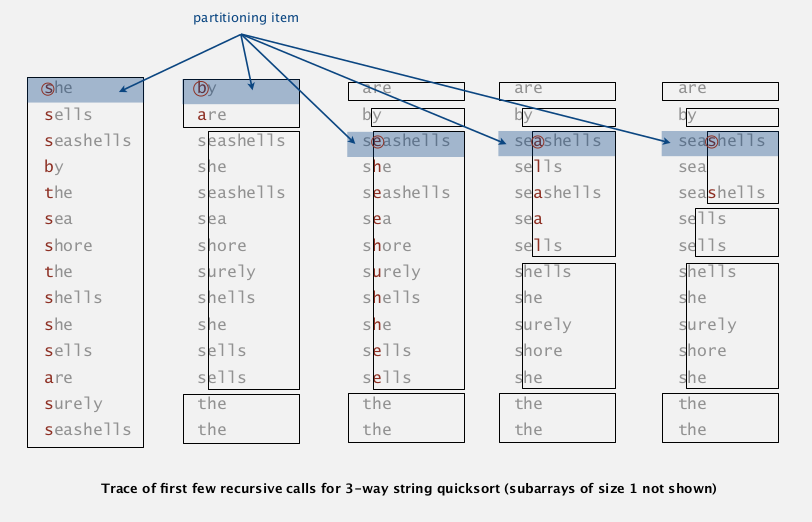### Implementation

modification of the 3-way qsort.

```private static sort(String[] a, int lo, int hi, int d){
if(hi<=lo) return;
int lt = lo, gt = hi, i=lo+1;// use 3 pointers: lt, i, gt
char pivot = a[lo].charAt(d);
while(i<=gt){//invariant: a[lo,lt)<pivot, a(gt,hi]>pivot, a[lt,i]=pivot
char c = a[i].charAt[d];
if(c<pivot) exch(a,lt++,i++);
else if(c>pivot) exch(a,i,gt--);
else: i++;
}//3-way partition
sort(a,lo,lt-1,d);
if(pivot>=0) sort(a,lt,gt,d+1);//pivot<0 means end-of-string
sort(a,gt+1,hi,d);
}
```

### analysis

wrt. qsort:

• from NlgN string compares to NlgN char compares.
• avoids re-comparing long common prefixes.

wrt. MSD:

• short inner loop
• cache friendly
• in-place# 6. Suffix Arrays

(some applications of suffix array)

given N chars (N huge), preprocess it to enable fast substring search.
⇒ suffix sort

• generate suffix array (linear time & space )
• sort on the suffix ⇒ brings repeated suffixes together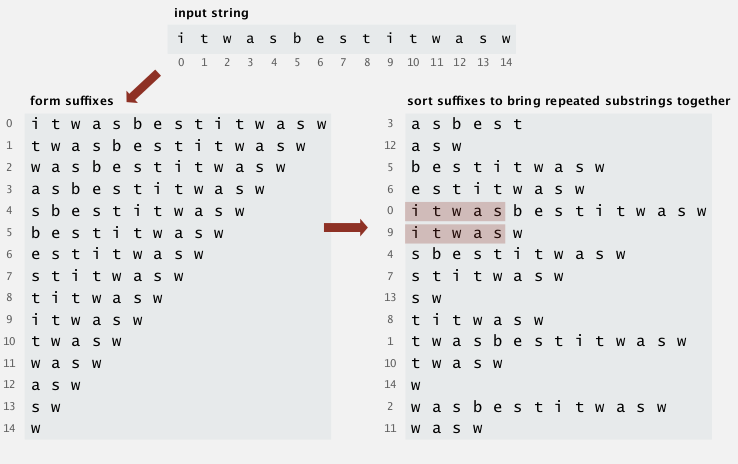### longest repeated substring

• brute force algorithm

try all i, j as starts of indices, then compute longest common prefix (LCP)
→ O(D*N2) where D is length of longest repeated substring.

• ⇒ use suffix array

sorting suffix array will bring repeated substrings together
java code:

```//int lcp(String s1, String s2) defined
public static lrs(String s){
int N = s.length;
String[] suffix = new String[N];
for(int i=0;i<N;i++) suffix[i] = s.substring(i);//construct suffix array
Arrays.sort(suffix);// sort suffix array ==> using 3-way radix sort
// one pass to get longest repeated substring (bigest lcp)
String lrs = "";
for(int i=0;i<N-1;i++){
int lcp = lcp(suffix[i], suffix[i+1]);
if(lcp>lrs.length) lrs = suffix[i].substring(0, lcp);
}
return lrs;
}
```

lrs worst-case input: lrs very long (say N/2).
quadratic for lrs and for sorting.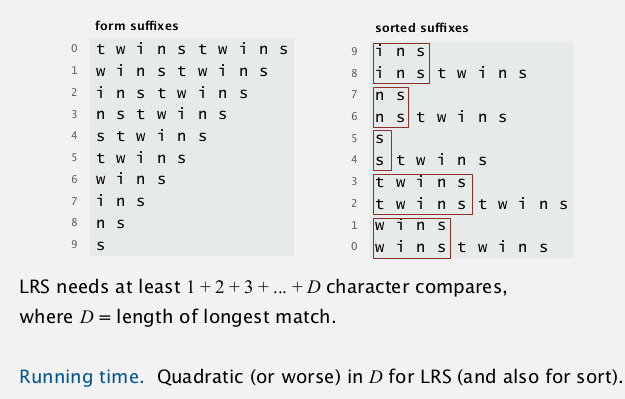improvement of lrs for worst-case performance:
Manber-Myers algo
sketch:

• phase 0: sort suffix[] on 1st char
• phase i: given suffix[] is sorted based on first 2^(i-1) chars → create suffix[] sorted on first 2^i chars.

each phase: double the nb of chars sorted on.
maintain an inverse[] array, to make comparisons constant time.
performance: NlgN

## String sorting summery

• can have linear sort: use chars as array index
• sublinear sort: not all date need to be examined
• 3-way radix qsort is asymptotically optimal